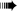1 Meter is equivalent to 100 Centimeter
Formula Used
1 Meter = 100 Centimeter
1 Meter = 100 Centimeter

## Interesting facts in MeterBurj Khalifa, the tallest building in the world stands at .Ostriches have a long small intestine.King Cobra is the biggest of all poisonous snakes and can grow to over long.

## Interesting facts in CentimeterThe largest land snail recorded was long.We are about taller in the morning than in the evening.On average, hair grows per year.

A
B
C
D
E
F
G
H
I
J
K
L
M
N
P
Q
R
S
T
V
X
Y

## Importance of Length converter

Measurement of various quantities has been an integral part of our lives since ancient times. In this modern era of automation, we need to measure quantities more so than ever. So, what is the importance of Length converter? The purpose of Length converter is to provide Length in the unit that you require irrespective of the unit in which Length was previously defined. Conversion of these quantities is equally important as measuring them. Length conversion helps in converting different units of Length. Length defines the most extended dimension of an object. There are various units which help us define Length and we can convert the units according to our requirement. UnitsConverters.com provides a simple tool that gives you conversion of Length from one unit to another.